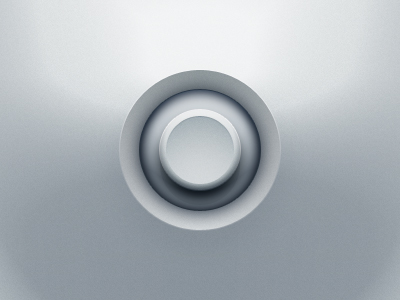There are several joystick scripts going around, however the few I stumbled upon had limited control sensitivity. A virtual analog controller should allow full 360 degree control (even if limited to certain directions within game-play, the system should allow for expansion/re-use), and MUST enable movement sensitivity (the character should move with a relative speed to the amount the joystick has moved).

In this post I am sharing a script I have written based on other implementations I have seen. Feel free to use it in your games.below is the script in action

This movie requires Flash Player 10

If you want to skin your joystick, here is a nice inspiration link
http://dribbble.com/shots/770444-Analog-Stick/rebounds

```package
{

import flash.events.Event;
import flash.events.MouseEvent;
import flash.display.MovieClip;

public class VirtualJoystick extends MovieClip
{

private var _startX:Number = 0;
private var _startY:Number = 0;
private var _tension:Number = .5;
private var _decay:Number = .7;
private var _xSpeed:Number = 0;
private var _isDragging:Boolean = false;
private var _angle:int;

public function VirtualJoystick()
{
_startX = joystick.x;
_startY = joystick.y;

}

//---------------------o
// Mouse down handler
//---------------------o
protected function on_mouseDown(e:MouseEvent):void
{
_isDragging = true;
}

//---------------------o
// Mouse up handler
//---------------------o
protected function on_mouseUp(e:MouseEvent):void
{
_isDragging = false;
}

//---------------------o
// EnterFrame handler
//---------------------o
protected function on_enterFrame(e:Event):void
{

if( _isDragging )
{

_angle = Math.atan2(root.mouseY-_startY,root.mouseX-_startX)/(Math.PI/180);

joystick.rotation = _angle;
joystick.knob.rotation = -_angle;

joystick.knob.x = joystick.mouseX;
}

//rotate player to match joystick direction
mcPlayer.rotation = _angle;

//keep player on screen
if( mcPlayer.y < 0 )
{
mcPlayer.y = 0;
}
if( mcPlayer.y > stage.stageHeight )
{
mcPlayer.y = stage.stageHeight;
}
if( mcPlayer.x < 0 )
{
mcPlayer.x = 0;
}
if( mcPlayer.x > stage.stageWidth )
{
mcPlayer.x =  stage.stageWidth;
}

//move player proportionate to amount of joystick movement
mcPlayer.y += Math.sin(_angle*(Math.PI/180))*(joystick.knob.x/8);
mcPlayer.x += Math.cos(_angle*(Math.PI/180))*(joystick.knob.x/8);

}
else{

//Return joystick to neutral position when not touching

_xSpeed = -joystick.knob.x*_tension;
joystick.knob.x += _xSpeed;

}

}
}
}
```

UPDATE:
I have had a few people asking me for the FLA for this example, so here it is:
http://www.johnstejskal.com/blog-files/joystick.rar